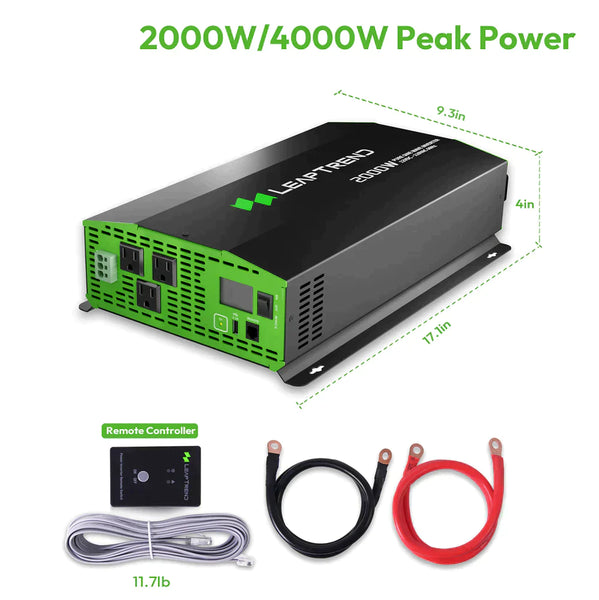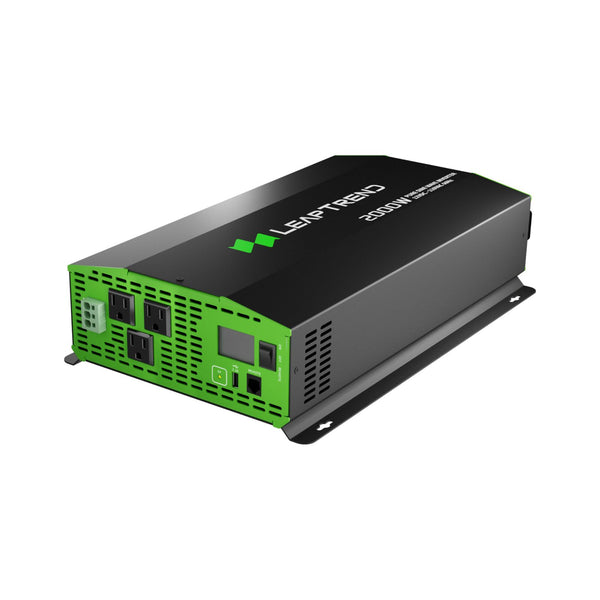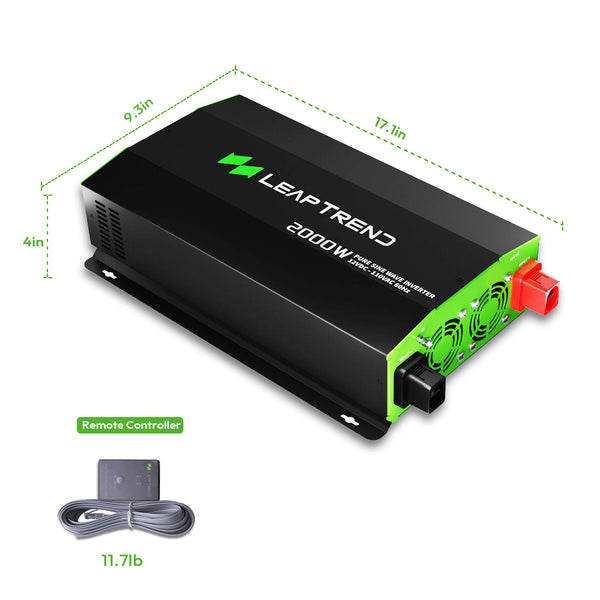# How Long Will a 2000W Inverter Run?

When it comes to using a 2000W inverter, understanding how long it can run is crucial for planning and optimizing power usage. In this article, we will explore the factors that affect the runtime of a 2000W inverter, provide calculations to estimate its duration, and offer some valuable tips to maximize its efficiency.

## Understanding a 2000W Inverter

A 2000W power inverter is a device that converts DC (direct current) power from a battery or other power source into AC (alternating current) power, allowing you to run various appliances and devices that require AC power. It is commonly used in RVs, boats, off-grid solar systems, and emergency backup power setups.

## How long does a 2000W inverter last?

If the inverter has a maximum load of 2000 watts and is running on a 200ah battery bank, it will stop in about an hour. When the current is higher, the battery loses power faster, so the inverter's run time is less than an hour.

​ If we are talking about the actual service life of the inverter, the warranty period is usually 10-15 years. However, a warranty doesn't tell the whole story.## Factors Affecting the Runtime of a power Inverter 2000W

### 1. Battery Capacity

The capacity of the battery connected to the inverter plays a significant role in determining its runtime. A higher-capacity battery can provide more energy, allowing the inverter to run for a longer duration.

### 2. Load or Power Consumption

The power consumption of the devices connected to the inverter, also known as the load, directly affects the runtime. Appliances with higher power requirements will drain the battery faster, reducing the overall runtime.

### 3. Efficiency of the Inverter

Inverters have an efficiency rating that indicates how effectively they convert DC power into AC power. Higher efficiency inverters will utilize the available energy more efficiently, resulting in a longer runtime.## Calculating the Runtime of a 2000w pure sine wave inverter

To estimate the runtime of a 2000w pure sine wave inverter, follow these steps:

### 1. Determine the Battery Capacity

Identify the capacity of the battery connected to the inverter, usually measured in ampere-hours (Ah) or watt-hours (Wh).

### 2. Calculate the Available Energy

Multiply the battery capacity (in Wh) by the battery voltage (in volts) to obtain the available energy in watt-hours.

### 3. Consider the Efficiency

Multiply the available energy by the efficiency of the inverter to account for any energy losses during the conversion process.

### 4. Divide by the Load

Divide the result by the power consumption of the connected devices (in watts) to estimate the runtime in hours.## Tips to Maximize the Efficiency of a 2000W Inverter 12V

### 1. Choose Energy-efficient Appliances

Opt for appliances and devices that have lower power requirements to reduce the load on the inverter and extend the runtime.

### 2. Optimize Power Usage

Use power-saving features on devices, turn off unnecessary appliances, and avoid running multiple high-power devices simultaneously to maximize the efficiency of the inverter.

### 3. Invest in High-quality Batteries

Choose high-capacity, deep-cycle batteries specifically designed for inverters to ensure longer runtimes and better overall performance.

In conclusion, the runtime of a 2000 watt inverter depends on factors such as battery capacity, power consumption of connected devices, and the efficiency of the inverter. By considering these factors and using the provided calculations, users can estimate the runtime of their 2000W inverter and make informed decisions about power usage. Following the tips mentioned in this article will help maximize the efficiency and extend the runtime of the inverter.